### Home > CALC > Chapter 8 > Lesson 8.1.6 > Problem8-62

8-62.
1.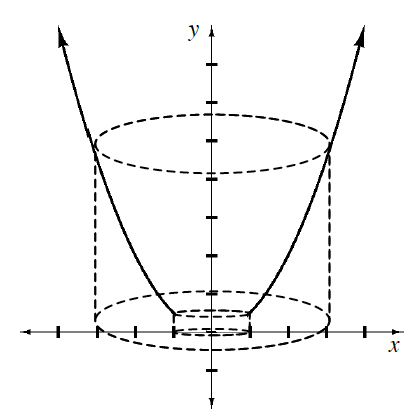The figure at right shows the region bounded by the x-axis, f(x) = 0.5x2 , x = l, and x = 3 . The region is revolved around the y-axis to create the solid shown with dotted lines. Homework Help ✎

1. Describe a method you can use to find the volume.

2. Setup the integrals and find the volume. (Using washers, the solution will require two integrals.)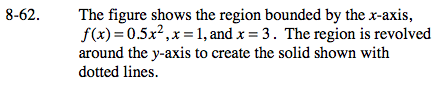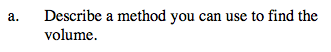Will you use Washers or Disks? Will the bounds and integrand be written in terms of x or y?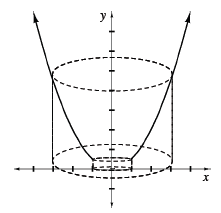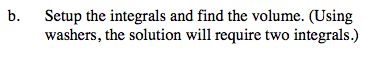The outside of this solid will be a cylinder with radius 3.

The inside of the solid will have a hole. But what shape is that hole? Is it cylindrical?

$\text{Or is it determined by }f(y) = \sqrt{(2y)}?$

Notice that the bottom of the hole is cylindrical (with radius 1), while the top of the hole is determined by

$f(y) = \sqrt{(2y)}.$

Consequently, you will need to use the Washer Method twice... both of which are rotated about the y-axis.

Use the eTool below to help solve the problem.
Click on the link to the right to view the full version of the eTool. Calc 8-62 HW eTool